# NanoPaleoMagnetism

A multiscale approach to paleomagnetic analysis of geological materials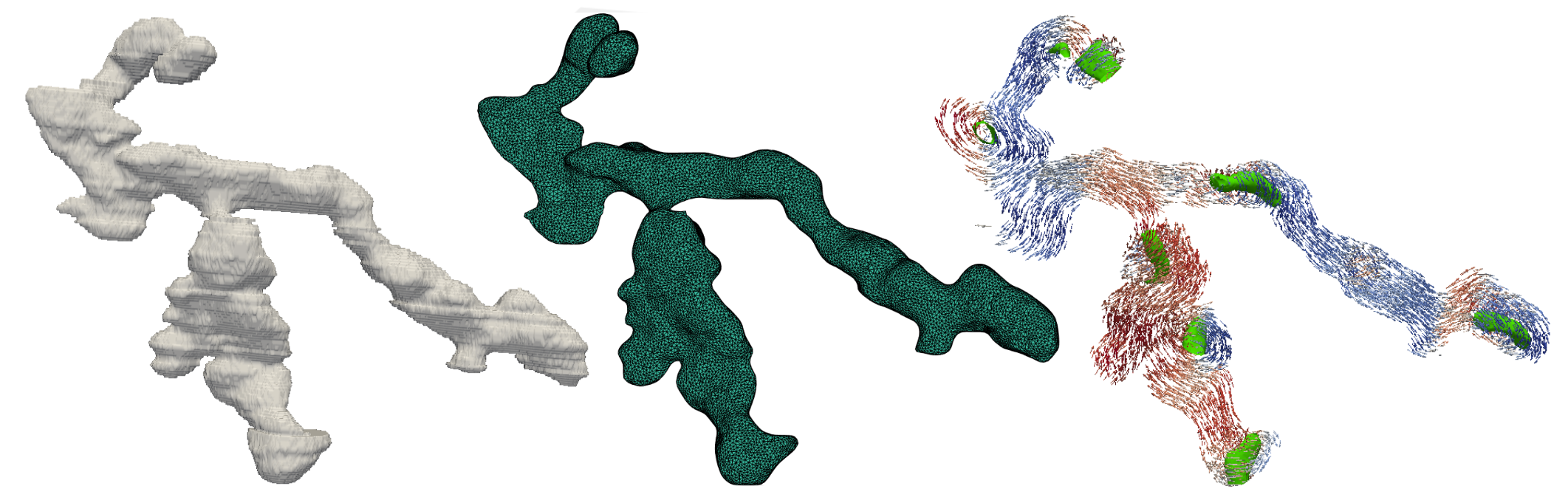## Mesh Generation – Iso2Mesh

This Guide is a Work In Progress

Iso2Mesh is an open source collection of functions which runs in either Matlab or Octave (an open source Matlab alternative) and can be used to create tetrahedral volume meshes of simple objects, cubes, ellipsoids, cylinders etc. or objects of any shape given a pre-existing surface mesh, which can be produced using Paraview. All examples shown here are produced in Matlab. Parameters should be defined in micrometers as these are the units used in MERRILL.

## Meshing Parameters

Iso2Mesh functions contain varying parameters which can be used to define the resolution of the resulting tetrahedral mesh produced. Care should be taken when using these parameters, since some control the surface mesh resolution, rather than the resolution throughout the mesh, which can lead to meshes which appear to have the desired resolution on the surface, but are much coarser within.

### The Opt Parameter

The opt parameter is used to define the surface resolution of a mesh. It can be defined in several ways;

• radbound defines the radius of the delauney sphere, which in turn defines the surface element size between the nodes.
• angbound defines the minimum angle (in degrees) of any surface triangle and therefore the shape of the surface facets.
• distbound defines the approximation error of the surface, it defines the maximum distance between the circumcenter of a surface facet and the centre of the Delauney sphere of this facet.

If opt is only defined as a scalar, this will define opt.radbound. The opt parameter should be defined alone before use in the meshing functions, rather than being defined with the inputs of the meshing functions.

### The Maxvol Parameter

Often the internal mesh resolution is defined by the use of the maxvol parameter, a scalar value which defines the target maximum volume of the internal tetrahedra of the mesh. The actual volume of the internal tetrahedra will vary depending on their shape, but this can be used to roughly define the internal element size using the formula for the volume of a regular tetrahedron:

Where V is the volume and a is the edge length.

### The Tsize Parameter

When creating simple objects, the surface element size is defined using the tsize parameter, a scalar which defines the maximum triangle size of the surface mesh.## Creating a Tetrahedral Volume Mesh## Simple Objects

Iso2Mesh contains several functions to create meshes of simple objects, spheres, ellipsoids, cuboids and cylinders. The user can define the dimensions of these objects along with the resolution of the produced mesh (using the meshing parameters discussed above).

### Spheres/Ellipsoids

Spheres and ellipsoids can be created using the ‘meshanellip’ function:

>> [node, face, elem] = meshanellip(c0, rr, tsize, maxvol);

Node, elem and face are the three output parameters of the tetrahedral mesh, node contains the coordinates of the nodes, face is an integer array containing the surface mesh elements and elem is an integer array containing the tetrahedral mesh elements within the volume of the object. If the function is used with the ‘elem’ parameter omitted, only a surface mesh will be produced.

• c0 defines the coordinates of the centre of the sphere/ellipsoid.
• rr can be defined in three ways. As a scalar, rr defines the radius of a sphere. As a 3 x 1 or 1 x 3 vector, rr defines the radii of the ellipsoid along x, y and z, whilst as a 5 x 1 or 1 x 5 vector, rr defines the radii along x, y and z as well as the angles, theta, the rotation axis along z and phi, the rotation axis along x.

Fig.1 shows an example ellipsoid mesh, both the surface and internal mesh, created using the input parameters; c0 = [0, 0, 0], rr = [0.08, 0.05, 0.05, pi/4, pi/4], tsize = 0.005, maxvol = 1.47e-08.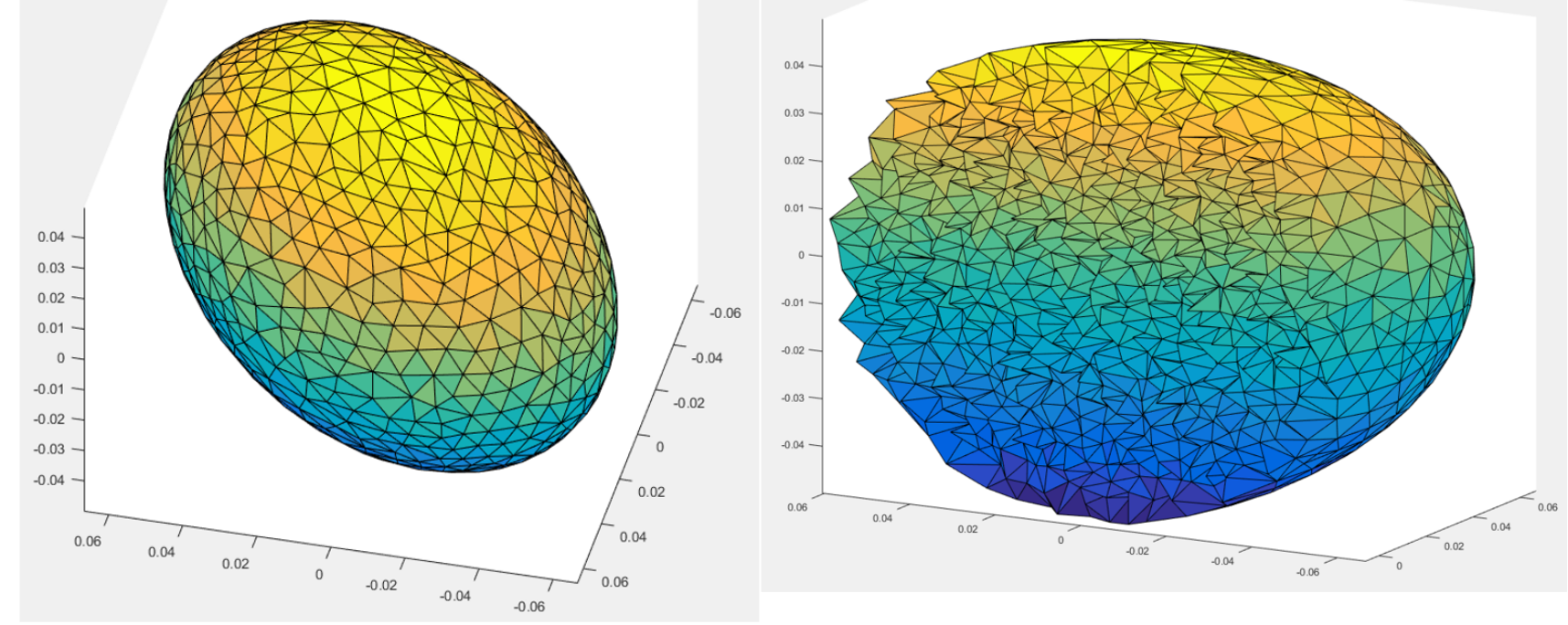Fig.1 shows both the surface (left) and internal (right) mesh of an ellipse created using the Iso2Mesh software

### Cuboids

Cuboids can be created using the ‘meshabox’ function:

>>[node, face, elem] = meshabox(p0, p1, maxvol, nodesize);

The outputs are node, elem and face, the same as above. The inputs however, are different from the mesh an ellipse function.

• p0 defines the coordinates of one corner of the cuboid.
• p1 defines the coordinates of the diagonally opposite corner of the cuboid to p0.
• nodesize is a parameter specific to the meshabox function, it defines the element size near to the vertices of the cuboid. Nodesize can be defined either as a single scalar or as a 8 x 1 array, defining the element size near to each vertex individually.

Fig.2 shows an example cuboid mesh, both the surface and internal mesh, created using p0 = [0, 0, 0], p1 = [0.08, 0.06, 0.04], maxvol = 1.47e-08 and nodesize = 0.005.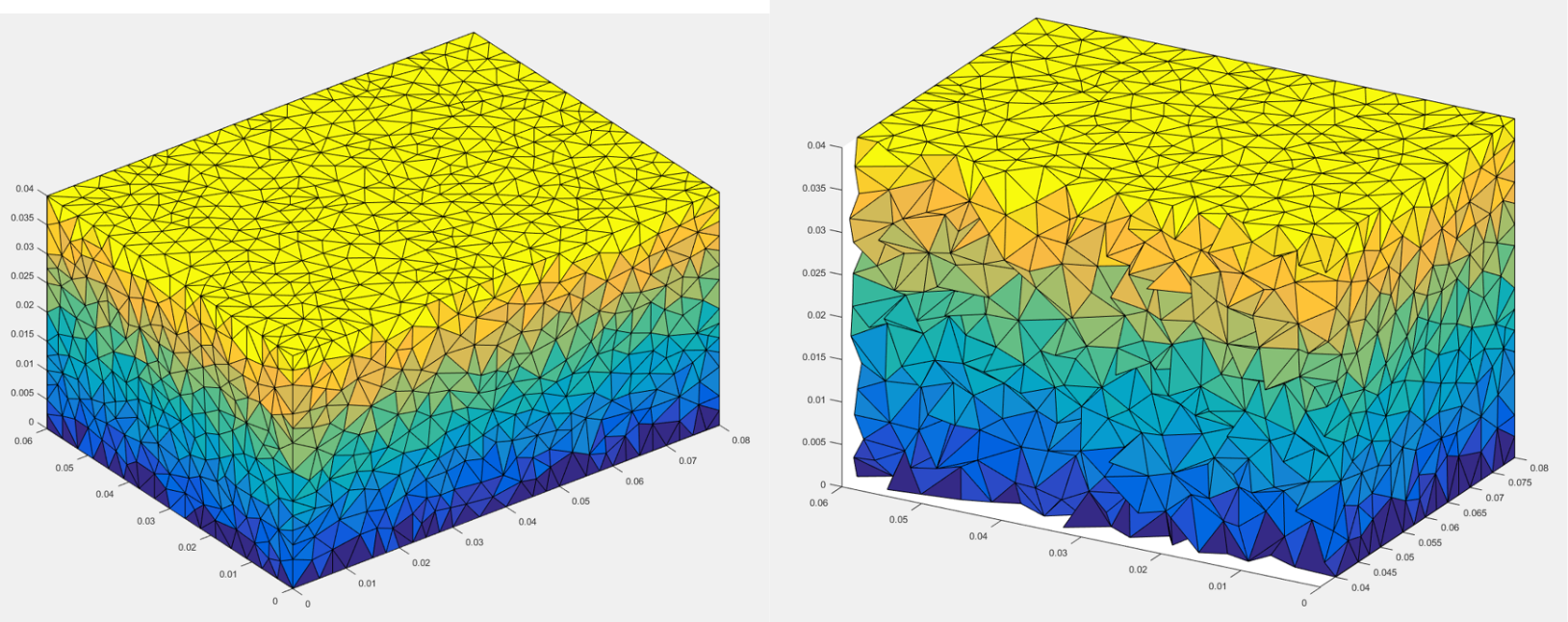Fig.2 shows the surface (left) and internal (right) mesh of a cuboid created using the Iso2Mesh software

### Cylinders

Cylinders can be created using the ‘meshacylinder’ function:

>>[node, face, elem] = meshacylinder(c0, c1, r, tsize, maxvol, ndiv);

The outputs are node, elem and face as above. The inputs however, are slightly different from the mesh and ellipse and mesh a box functions.

• c0 defines the coordinates of one end of the cylinder.
• c1 defines the coordinates of the other end of the cylinder to c0.
• r defines the radius of the cylinder.
• ndiv defines the number of flat surfaces which the curved cylindrical surface will be approximated by, if no value of ndiv is input, a value of 20 will be used.

Fig.3 shows an example cylindrical mesh, both the surface and internal mesh, created using c0 = [0, 0, 0], c1 = [0, 0, 0.08], r = 0.02, tsize = 0.005, maxvol = 1.47e-08 and ndiv = 30.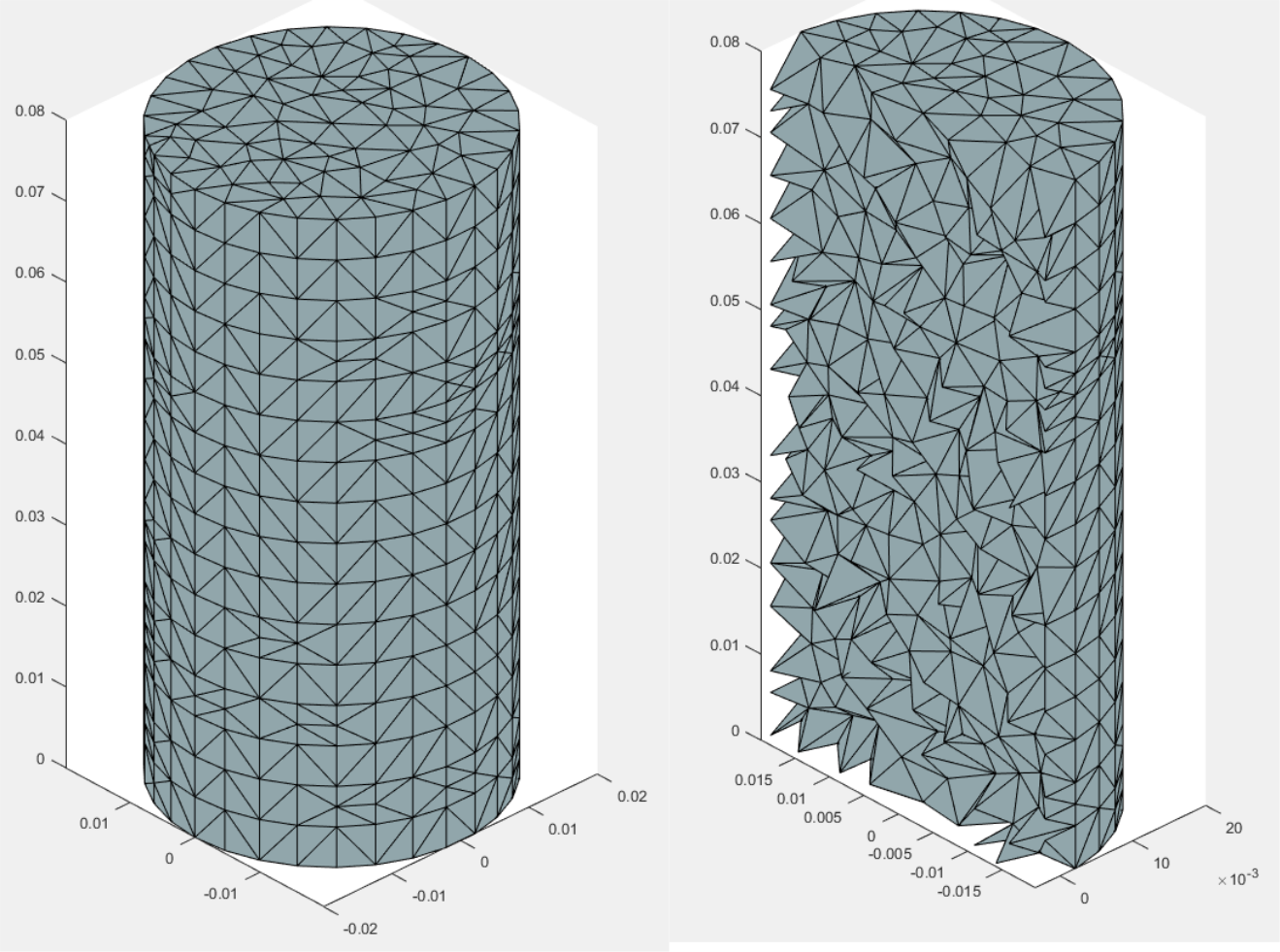Fig.3 shows both the surface (left) and internal (right) mesh of a cylinder made using the Iso2Mesh software## Objects From Image Stacks

Objects contained within .tiff (or other image stack formats) can also be meshed using Iso2Mesh. This requires the data to be converted to a surface using Paraview and imported to the Iso2Mesh directory using the ‘stlread’ function, which can be found at the top of this page.

### Paraview

Using our data visualisation guide, found here https://nanopaleomag.esc.cam.ac.uk/?page_id=1730 , image stack data can be loaded into Paraview and the aspect ratio/voxel size can be defined. Once this is done a surface can be taken using the contour function, details of which can again be found in the aforementioned guide. This surface can then be saved off as a .stl file as shown in Fig.4.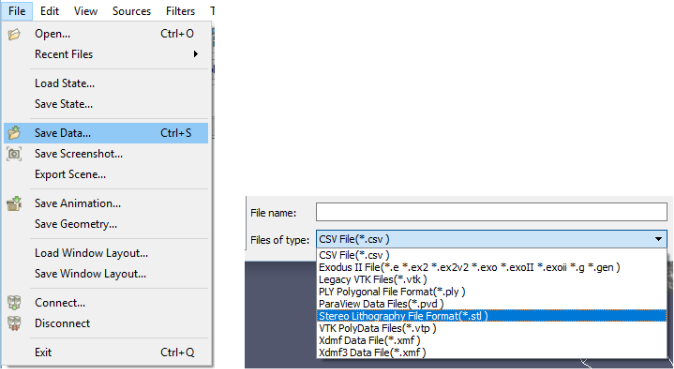Fig.4 shows the Save Data function (left) and the file type to save the surface as, .stl (right)

### Iso2Mesh

In order to open the .stl surface into Matlab/Octave, both the file containing the stlread function and the .stl surface file must be placed in the same directory as the Iso2Mesh files. Once this has been done the surface can be loaded using the stlread function:

The outputs of this function are f and v, f contains the faces, whilst v contains the vertices of the surface mesh. Once the surface mesh has been loaded into this format the volume mesh can be created, using the ‘cgals2m’ function:

>> [node, elem, face] = cgals2m(v, f, opt, maxvol);

The outputs of this function are node, elem and face, as in the simple mesh examples above. The inputs are the vertices and faces of the surface mesh (v and f respectively), opt and maxvol. Fig.5 shows an example mesh created using a smoothed version of the example particle as used in the data visualisation guide and input parameters: opt = 0.01, maxvol = 1.17e-02.

## Saving Meshes for MERRILL

Currently, tetrahedral volume meshes can be saved using the ‘merrillsave’ function, written and provided by Dr Karl Fabian, which is available on request.

>> merrillsave(‘filename’, node, elem);